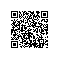# 【哈夫曼编码】HDU2527-Safe Or Unsafe

Safe Or Unsafe
Time Limit: 2000/1000 MS (Java/Others)    Memory Limit: 32768/32768 K (Java/Others)
Total Submission(s): 1474    Accepted Submission(s): 582

Problem Description
Javac++ 一天在看计算机的书籍的时候，看到了一个有趣的东西！每一串字符都可以被编码成一些数字来储存信息，但是不同的编码方式得到的储存空间是不一样的！并且当储存空间大于一定的值的时候是不安全的！所以Javac++ 就想是否有一种方式是可以得到字符编码最小的空间值！显然这是可以的，因为书上有这一块内容--哈夫曼编码(Huffman Coding)；一个字母的权值等于该字母在字符串中出现的频率。所以Javac++ 想让你帮忙，给你安全数值和一串字符串，并让你判断这个字符串是否是安全的？

Input

Output

Sample Input
2
12
helloworld
66
ithinkyoucandoit

Sample Output
no
yes

Source
HDU 2008-10 Programming Contest

AC代码：

#include<stdio.h>
#include<string.h>
#include<algorithm>
#include<queue>
using namespace std;
char a;
int flag;
int main()
{
int i,j,n,m,k,x,y,sum;
scanf("%d",&n);
while(n--)
{
scanf("%d",&m);
memset(a,0,sizeof(a));
scanf("%s",&a);
k=strlen(a);
memset(flag,0,sizeof(flag));
for(i=0;i<k;i++)
{
flag[a[i]-'a']++;
}

priority_queue<int,vector<int>,greater<int> > q;
for(i=0;i<26;i++)
{
if(flag[i]!=0)
q.push(flag[i]);
}
sum=0;
if(q.size()==1)
{
if(k>m)
printf("no\n");
else
printf("yes\n");
}
else
{
while(q.size()>1)
{
x=q.top();q.pop();
y=q.top();q.pop();
sum+=(x+y);
q.push(x+y);
}

if(sum<=m)
printf("yes\n");
else
printf("no\n");
}
}
return 0;
}


3
12
helloworld
x=1 y=1 sum=2
x=1 y=1 sum=2
x=1 y=2 sum=3
x=2 y=2 sum=4
x=3 y=3 sum=6
x=4 y=6 sum=10
sum=27
no
66
ithinkyoucandoit
x=1 y=1 sum=2
x=1 y=1 sum=2
x=1 y=1 sum=2
x=1 y=2 sum=3
x=2 y=2 sum=4
x=2 y=2 sum=4
x=2 y=3 sum=5
x=3 y=4 sum=7
x=4 y=5 sum=9
x=7 y=9 sum=16
sum=54
yes使用钉钉扫一扫加入圈子
+ 订阅